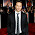### SYNCHRONOUS MACHINE TERMINAL VOLTAGE TRANSDUCER AND LOAD COMPENSATOR MODELS TUTORIALS

A block diagram of the terminal voltage transducer and the load compensator is shown in Fig 2. These model elements are common to all excitation system models described in this document.

Figure 2-Terminal Voltage Transducer and Optional Load Compensation Elements

It is realized that, for some systems, there may be separate and different time constants associated with the functions of voltage sensing and load compensation. The distinction is not recognized in this model, in which only one time constant, TR, is used for the combined voltage sensing and compensation signal.

When load compensation is not employed (RC=XC= 0), the block diagram reduces to a simple sensing circuit. The terminal voltage of the synchronous machine is sensed and is usually reduced to a dc quantity.

While the filtering associated with the voltage transducer may be complex, it can usually be reduced, for modeling purposes, to the single time constant, TR, shown. For many systems, this time constant is very small, and provision should be made to set it to zero.

The terminal voltage transducer output, VC, is compared with a reference that represents the desired terminal voltage setting, as shown on each of the excitation system models. The equivalent voltage regulator reference signal, VREF, is calculated to satisfy the initial operating conditions.

It will, therefore, take on a value unique to the synchronous machine load condition being studied. The resulting error is amplified as described in the appropriate excitation system model to provide the field voltage and subsequent terminal voltage to satisfy the steady-state loop equations.

Without load compensation, the excitation system, within its regulation characteristics, attempts to maintain a terminal voltage determined by the reference signal. When compensation is desired, the appropriate values of RC and XC are entered.

In most cases, the value of RC is negligible. The input variables of synchronous machine voltage and current must be in phasor form for the compensator calculation. Care must be taken to ensure that a consistent per unit system is utilized for the compensator parameters and the synchronous machine current base.

This type of compensation is normally used in one of the following two ways:
1) When units are bused together with no impedance between them, the compensator is used to create an artificial coupling impedance so that the units will share reactive power appropriately. This corresponds to the choice of a regulating point within the synchronous machine. For this case, RC and XC would have positive values.

2) When a single unit is connected through a significant impedance to the system, or when two or more units are connected through individual transformers, it may be desirable to regulate voltage at a point beyond the machine terminals.

For example, it may be desirable to compensate for a portion of the transformer impedance and effectively regulate voltage at a point part way through the step-up transformer. For these cases,
RC and XC would take on the appropriate negative values.

Some compensator circuits act to modify terminal voltage as a function of reactive and real power, instead of reactive and real components of current. Although the model provided will be equivalent to these circuits only near rated terminal voltage, more precise representation has not been deemed worthwhile.

1.2.